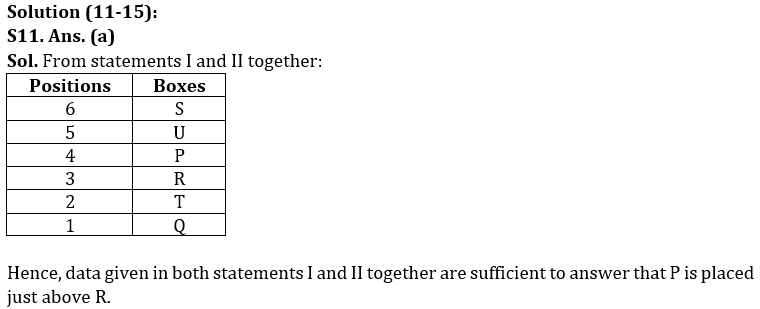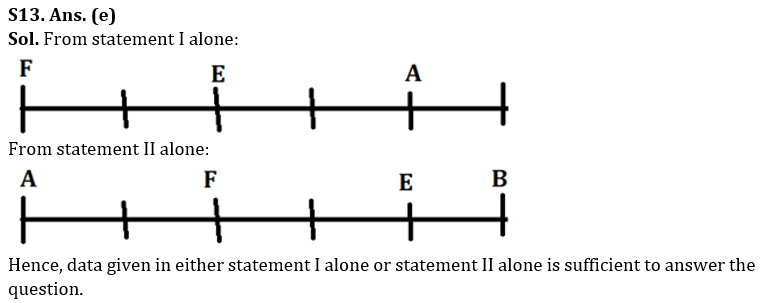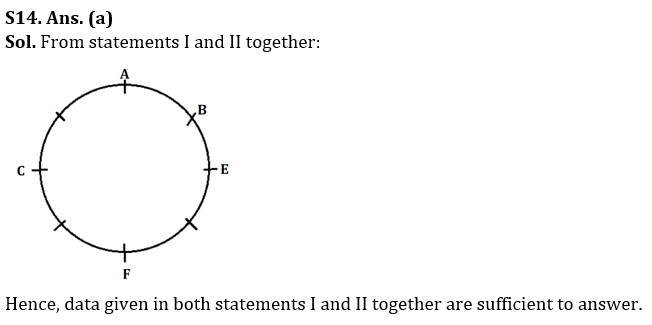Latest Banking jobs   »

# Reasoning Ability Quiz For LIC ADO Mains 2023-27th March

Directions (1-5): A word arrangement machine when given an input of words, rearranges the words following a particular set of rules. An illustration of that is given below.

Input: RAM TYU GTR WTI VFD DGH
Step I: DGH GTR RAM TYU VFD WTI
Step II: DGH GRT AMR TUY DFV ITW
Step III: EHI HSU ZNS UTZ EGW HUX
Step IV: ZNS UTZ HUX HSU EHI EGW
Step V: NSZ TUZ HUX HSU EHI EGW

Step V is the last step of the rearrangement. Following the same set of rules rearrange the input given below and answer the questions based on it.

Input: PQU GRB UTV XQJ WYK SHD

Q1. Which of the following is the last step of the given input?
(a) TUW QRT LXZ KRY EIT CHS
(b) TUW LXZ QRT KRY EIT CHS
(c) TUW QRT LXZ EIT KRY CHS
(d) TUW LXZ QRT KRY CHS EIT
(e) None of these

Q2. Which word is the third from the right end in step III of the given input?
(a) EIT
(b) UTW
(c) QRT
(d) LXZ
(e) None of these

Q3. In which step ‘QRT EIT UTW’ will be found in the same sequence?
(a) Step II
(b) Step IV
(c) Step III
(d) Step V
(e) None of these

Q4. What is the position of ‘QRT’ from right end in the step IV of the given input?
(a) First
(b) Fourth
(c) Fifth
(d) Second
(e) None of these

Q5. Which of the following is the second last step of the given input?
(a) UTW QRT LXZ KRY EIT CHS
(b) TUW QRT LXZ KRY EIT CHS
(c) TUW LXZ QRT KRY CHS EIT
(d) UTW QRT LXZ EIT KRY CHS
(e) None of these

Directions (6-10): A six digits number arrangement machine when given an input line of numbers rearranges them following a particular rule in each step. The following is an illustration of input and rearrangement.

Input: 785645 574831 931685 314876 613295 416793
Step I: 774835 585641 914875 331686 616795 413293
Step II: 1609 1226 1789 1017 1411 706
Step III: 3528 3445 3678 3236 3633 625
Step IV: 18 16 24 14 15 13
Step V: 54 48 72 42 60 52
Step VI: @# ## @# ## ## @#

Step VI is the last step of the above input. As per rules followed in the above steps, find out in each of the given questions the appropriate steps for the given input.

Input: “832719  523847  265793  731546  462753  183452”

Q6. Which of the following number is 2nd from the left side in step III?
(a) 855
(b) 3753
(c) 3565
(d) 3468
(e) None of these

Q7. What is the sum of the first and the last numbers in step V of the given input?
(a) 150
(b) 122
(c) 113
(d) 120
(e) None of these

Q8. What is the sum of the all digits of the number, which is 3rd from the right end in step IV of the given input?
(a) 17
(b) 8
(c) 6
(d) 9
(e) None of these

Q9. How many # are there in the final step?
(a) 5
(b) 6
(c) 2
(d) 8
(e) None of these

Q10. What is the product of 1st and 4th digit of a number, which is 4th from the left end in step I (digits are taken from the left end)?
(a) 49
(b) 48
(c) 58
(d) 64
(e) None of these

Directions (11-15): The question consists of two statements numbered I and II given below it. You have to decide whether the data provided in the statement is sufficient to answer the question.

Q11. Six boxes, P, Q, R, S, T and U are placed one above another, where the bottommost box is 1, the box above it is 2 and so on up to 6 which is placed at the topmost position. Which box ix placed just above R?
Statements: I. P is placed 2nd above of T which is placed just above of Q. Two boxes are placed between T and U.
II. S is placed above P. R is not placed at the even numbered position.
(a) Data given in both statements I and II together are sufficient to answer.
(b) Data given in statement I alone is sufficient to answer.
(c) Data given in statement II alone is sufficient to answer.
(d) Data given in both statements I and II together are not sufficient to answer.
(e) Data given in either statement I or statement II alone is sufficient to answer the question.

Q12. There are six persons namely, A to F of different ages. Who is the oldest person?
Statements: I. E is older than A who is older than C. F is younger than D.
II. D is older than E. B is not the oldest person.
(a) Data given in both statements I and II together are sufficient to answer.
(b) Data given in statement I alone is sufficient to answer.
(c) Data given in statement II alone is sufficient to answer.
(d) Data given in both statements I and II together are not sufficient to answer.
(e) Data given in either statement I or statement II alone is sufficient to answer the question.

Q13. Who sits second to the right of F if A, B, C, D, E and F are sitting in a straight row facing north but not necessarily in the same order?
Statements: I. Three persons sit between A and F who sits at the extreme left end of the row. A sits second to the right of E.
II. B sits third to the right of F who sits second to the right of A. Three persons sit between A and E.
(a) Data given in both statements I and II together are sufficient to answer.
(b) Data given in statement I alone is sufficient to answer.
(c) Data given in statement II alone is sufficient to answer.
(d) Data given in both statements I and II together are not sufficient to answer.
(e) Data given in either statement I alone or statement II alone is sufficient to answer the question.

Q14. Eight persons A, B, C, D, E, F, G, and H are sitting along a circular table facing towards the center of the table but not necessarily in the same order. Who sits second to the right of A?
Statements: I. Three persons sit between A and F who sits second to the left of E.
II. B sits third to the right of F who sits second to the right of C.
(a) Data given in both statements I and II together are sufficient to answer.
(b) Data given in statement I alone is sufficient to answer.
(c) Data given in statement II alone is sufficient to answer.
(d) Data given in both statements I and II together are not sufficient to answer.
(e) Data given in either statement I alone or statement II alone is sufficient to answer the question.

Q15. Eight persons P, Q, R, S, T, U, V, and W are sitting around a circular table facing the center. Who sits to the immediate right of W?
Statements: I. P sits third to the right of U. W sits second to the right of P.
II. R does not sit adjacent to S.
(a) Data given in both statements I and II together are sufficient to answer.
(b) Data given in statement I alone is sufficient to answer.
(c) Data given in statement II alone is sufficient to answer.
(d) Data given in both statements I and II together are not sufficient to answer.
(e) Data given in either statement I alone or statement II alone is sufficient to answer the question.

Solutions

Solution (1-5):
Sol.

Step I: The words are arranged in the alphabetical order from the left end
Step II: The letters of the words are arranged in alphabetical order within the word.
Step III: The consonants are replaced with the next letter and the vowels are replaced with the previous letter of the English alphabetical series (within each word).
Step IV. The words are arranged in reverse alphabetical order from left end.
Step V: The letters of the word are arranged in alphabetical order within the word.

Input: PQU GRB UTV XQJ WYK SHD
Step I: GRB PQU SHD UTV WYK XQJ
Step II: BGR PQU DHS TUV KWY JQX
Step III: CHS QRT EIT UTW LXZ KRY
Step IV: UTW QRT LXZ KRY EIT CHS
Step V: TUW QRT LXZ KRY EIT CHS

S1. Ans.(a)
S2. Ans.(b)
S3. Ans.(c)
S4. Ans.(c)
S5. Ans.(a)

Solution (6-10):
Sol. Different logic is applied in each step.
Step I: middle four digits of first number are interchanged by middle four digits of the second number. Similarly, middle four digits of third number are interchanged by middle four digits of the fourth number. This process continues with fifth and sixth number.
Step II: First break the number in two parts: 1st number = first three digits and 2nd number = last three digits of the original number. Then combine both 1st and 2nd number and get the resultant number in step II.
Step III: One is subtracted from the digits which are greater than 5 and 2 is added to the digits which are less than or equal to 5.
Step IV: sum of the digits is written.
Step V: odd numbers are multiplied by 4 whereas even numbers are multiplied by 3
Step VI: we write @, If the digit is odd and we write # if the digit is even.

Input: 832719  523847  265793  731546  462753  183452
Step I: 823849 532717 231543 765796 483453 162752
Step II: 1672 1249 774 1561 936 914
Step III: 3564 3468 666 3753 855 836
Step IV: 18 21 18 18 18 17
Step V: 54 84 54 54 54 68
Step VI: @# ## @# @# @# ##

S6. Ans. (d)
S7. Ans. (b)
S8. Ans. (d)
S9. Ans. (d)
S10. Ans. (a)S12. Ans. (a)
Sol. From statements I and II together: D > E > A > C and D > F
Hence, data given in both statements I and II together are sufficient to answer that D is the oldest person.S15. Ans. (d)
Sol. From both statements, we can’t get the answer. So, data given in both statements I and II together are not sufficient to answer.## FAQs

### When is the LIC ADO Mains Exam scheduled?

The LIC ADO Mains exam is scheduled to be held on 23 April 2023

#### Congratulations!Union Budget 2023-24: Free PDF